# Logic facts for kids

Kids Encyclopedia Facts
(Redirected from Logical)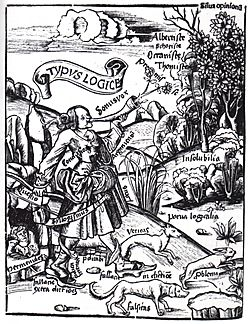Gregor Reisch, Logic presents its main themes. Margarita Philosophica, 1503 or 1508. Two dogs veritas (truth) and falsitas (falsehood) chase a rabbit called problema. Logic runs behind the dogs, armed with the sword syllogismus. In the bottom left corner, the philosopher Parmenides can be seen in a cave.

Logic is the science of reasoning. Logic helps people decide whether something is true or false.

A popular example of a syllogism given by Aristotle:

1. All men are mortal
2. Socrates is a man
3. Therefore, Socrates is mortal.

## Symbolic logic

The same syllogism can be written in a notation: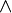$\land$ is read like "and", meaning both of the two.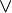$\lor$ is read like "or", meaning at least one of the two.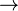$\rightarrow$ is read like "implies", or "If ... then ...".$\lnot$ is read like "not", or "it is not the case that ...". Parentheses (,) are added for clarity and precedence; this means that what is in parenthesis should be looked at before the things outside.

This is the same example using logic symbols: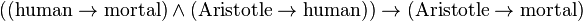$\rm((human\rightarrow mortal)\land(Aristotle\rightarrow human))\rightarrow(Aristotle\rightarrow mortal)$

And this is the same example using general terms: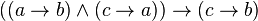$((a\rightarrow b)\land(c\rightarrow a))\rightarrow(c\rightarrow b)$

Finally, those talking about logic talk about statements. A statement is simply something like "Aristole is human" or "all humans are mortal". Statements have a truth value; they are either true or false, but not both. Mistakes in logic are called "fallacies".

## Logical proof

A logical proof is a list of statements. Each statement in the proof is either an assumption or has been proven to follow from earlier statements in the proof. A proof shows that one statement, the conclusion, follows from the assumptions. One can, for example, prove that "Aristotle is mortal" follows from "Aristotle is a man" and "All men are mortal".

There are statements that are always true.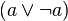$(a \lor \lnot a)$ is always true. It is called a tautology. (for example: "Either it rains, or it does not rain")

## Uses

Logic is used by computers in what is called an algorithm. An algorithm is sort of like a cooking recipe; it tells the computer what to do and when to do it.

Logic is used in mathematics. People who study math create proofs that use logic to show that math facts are correct. There is an area of mathematics called mathematical logic that studies logic using mathematics.

Logic is also studied in philosophy.

## Images for kidsLogic Facts for Kids. Kiddle Encyclopedia.